# About the Constraints Satisfaction Editor

The dialog box is displayed when you click the Constraint Satisfaction (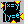) icon. It is made up of three tabs.

## Editors tab

The Editor is divided into 3 main fields:

• The constraints body, i.e. their textual representation
• The Inputs and Outputs of the constraints
• The Dictionary of parameters and functions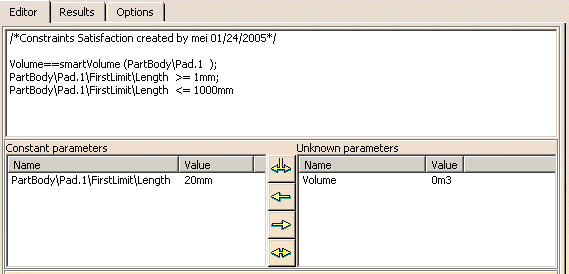Constant parameters: The values of constant parameters are set by you and are considered as constant by the solver. This value can be changed directly in the Value column by clicking twice (slowly) in the Value cell. Unknown parameters: The value of unknown parameters will be calculated after you push the Solve button.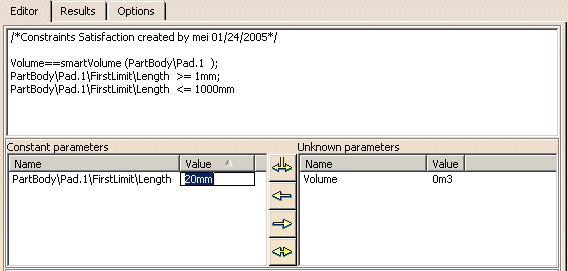Note that you can edit the value of the constant parameters by clicking the value in the value field. (See picture opposite.)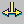The Parse arrow is used to identify the variables of the set of constraints. It must be pushed before choosing input and output variables.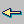The left arrow is used to move variables from the Unknown parameters category to the Constant parameters one.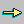The right arrow is used to move variables from the Constant parameters category to the Unknown parameters one.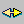The Switch input/output arrow is used to swap the selected constant and unknown parameters.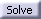Click Solve to launch the computation.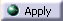Click Apply to launch the update of the model.

## Results tab

Note that you can now find more than one solution to a single problem. To get an example, see Using Measured Parameters in a Constraint Satisfaction Computation.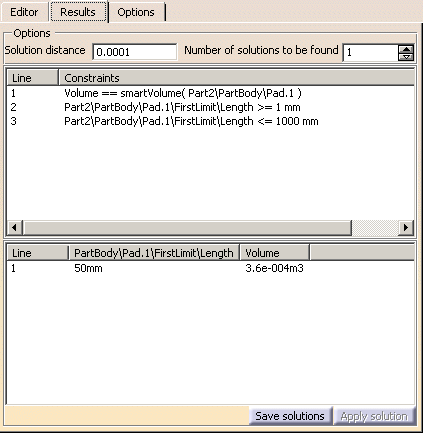Solution Distance: Enter the distance between the different solutions that you want to find. Number of solutions to be found: Enter the number of solutions to be found.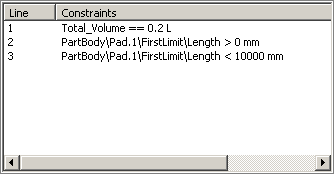This field displays each constraint identifier in the body.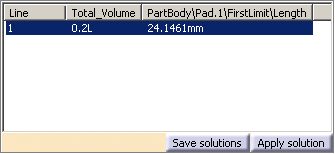This field displays the parameters taken into account by the computation and shows the solution found.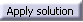Select one of the solutions and click Apply solution. The solution is automatically applied to the geometry and the parameters are valuated with those of the solution.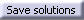Click this button if you want to save the result of the computation. Note that the results of the computation can be saved either in .txt or .xls format.

## Options tab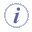Black box refers to a measure function. For instance, smartVolume is a measure that calculates the volume of a body.
Algorithm
• Precision: Enables you to define the precision of the results (i.e the number of decimal digits after the decimal point.) This precision is used when comparing 2 numbers. This option defines whether they are equal or not. When dealing with units, the comparison is made on the basis of the MKS value of parameters.

Note that if the precision is set to 0 the precision is set to 1e-10. The black box precision is set to 1e-11. This may result in a very long computation time to solve the set of equations.
• Use Gauss method for linear equations: Accelerates the solve operation when working with linear equations.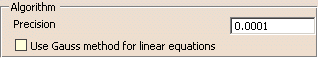Termination criteria
• Maximum computation time (sec.): Enables you to indicate the computation time. If the indicated time is equal to 0, the computation will last until a solution is found.
• Show 'Stop' dialog: If checked, displays a "Stop" dialog box that will enable you to interrupt the computation.
• Generate expanded error description: Enables you to get detailed information about errors if they occur.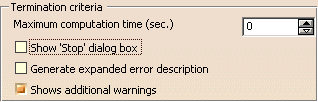Note that the dialog box STOP is displayed only after a few seconds. If the computation is performed quickly, it is not displayed.

Black boxes algorithm: You have the choice between 2 algorithms. If your problem behaves like quadratic functions, select Quadratic approximation.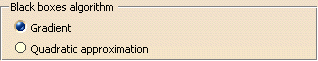Black boxes parameters
• Precision (for black boxes): A real number taken from the interval [1e- 10, 0,1]. This number has an influence on the accuracy of the solution found.
• Maximal number of black boxes calls: This option allows you to limit the number of measures calculation. (equivalent to a time limit.)
• Number of attempts: This option is only used for sets of constraints with more than one variable. This option changes the number of start points used by the solver. For instance, for a 2-dimensional problem, the following number of calls will be performed:
Number of attempts: 1- > 3 start points
Number of attempts: 2- > 9 start points
Number of attempts: 3- > 27 start points
• Use unimodal interval: This option allows you to use a special information about the interval of unimodality.
• Minimize the number of black boxes calls: If checked:
 Enables to minimize the number of black boxes evaluations. If you need several solutions, the fact that the solutions found will be close to each other is not guaranteed.

In both cases (option checked or not), solutions will be found.

• Width: This parameter sets the minimal width of the interval of unimodality for measures. For linear, quadratic and any monotonic measure, this value can be set to 1e 19. In a common case, the greater this value, the quicker the solver finds the solutions. However if you are not sure about the monotonicity of the problem, do not use this option.
• Cache size: This option allows you to choose the size of internal black boxes caches. Decrease this value only if you do not have enough memory. Increase this value if you have enough memory to reduce the computation time.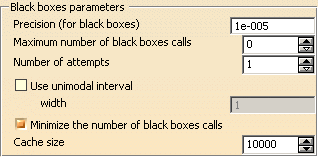#### Error and Warning messages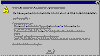Unsensitive measures to unknown parameters window (click the graphic opposite to enlarge it). This dialog box is displayed when a measure is not sensitive to a variation of any unknown parameter. It is usually the case when: The measure is really independent from the unknown variables. (You must replace this measure with a constant if you are sure that this is the case, it will speed up the computation.) The black box precision is too low: The measures are not sensitive to a small variation of the unknown variables. You can increase the black box precision option.